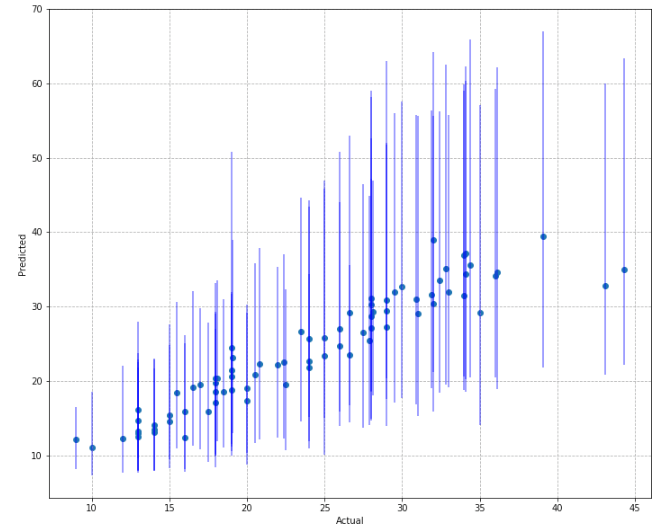# Estimating Uncertainty in Machine Learning Models — Part 3

Source: Deep Learning on Medium# Estimating Uncertainty in Machine Learning Models — Part 3

Check out part 1 (here)and part 2 (here) of this series

In the last part of our series on uncertainty estimation, we addressed the limitations of approaches like bootstrapping for large models, and demonstrated how we might estimate uncertainty in the predictions of a neural network using MC Dropout.

So far, the approaches we have looked at have involved creating variations in the dataset, or the model parameters to estimate uncertainty. The main drawback here is that it requires us to either train multiple models, or make multiple predictions in order to figure out the variance in our model’s predictions.

In situations with latency constraints, techniques such as MC Dropout might not be appropriate for estimating a prediction interval. What can we do to reduce the number of predictions we need to estimate the interval?

# Using Maximum Likelihood Method (MLE) to estimate Intervals

In part 1 of this series, we made an assumption that the mean response of our dependent variable, μ(y|x), is normally distributed.

The MLE method involves building two models, one to estimate the conditional mean response, μ(y|x) , and another to estimate the variance, σ² in the predicted response.

We do this by first, splitting our training data into two halves. The first half model, is trained as a regular regression model, using the first half of the data. This model is then used to make predictions on the second half of the data.

The second model, mσ² is trained using the second half of the data, and the squared residuals of as the dependent variable.